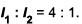Courses

# Inteference NAT Level - 2

## 10 Questions MCQ Test Topic wise Tests for IIT JAM Physics | Inteference NAT Level - 2

Description
This mock test of Inteference NAT Level - 2 for Physics helps you for every Physics entrance exam. This contains 10 Multiple Choice Questions for Physics Inteference NAT Level - 2 (mcq) to study with solutions a complete question bank. The solved questions answers in this Inteference NAT Level - 2 quiz give you a good mix of easy questions and tough questions. Physics students definitely take this Inteference NAT Level - 2 exercise for a better result in the exam. You can find other Inteference NAT Level - 2 extra questions, long questions & short questions for Physics on EduRev as well by searching above.
*Answer can only contain numeric values
QUESTION: 1

### A Michelson interferometer is configured as a wave meter as shown in the figure below may be used to compare the wavelengths of 2 laser with high precision when the mirror is the right arm of the interferometer is translated through a distance d, 1,00,000 interference fringes pass across the detector for green light and 85,865 fringes pass across the detector for red (λ = 632.82 nm) light. What is the wavelength of the green laser light in nm?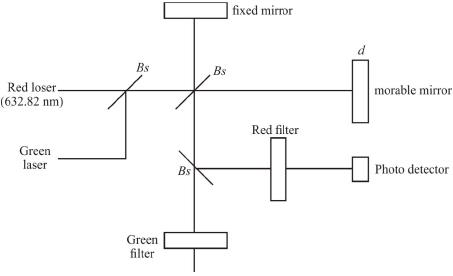Solution:

A fringe shift occurs whenever  d = λ
Thus,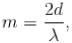where m is the number of fringes &  λ is the wavelength
For red beam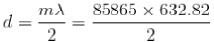For green beam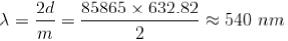*Answer can only contain numeric values
QUESTION: 2

### Light from a laser falls on a pair of very narrow slits separated by 0.5 μm & bright fringes separated by 1.0 mm are observed on a distant screen if the frequency of the laser light is doubled, how much times the separation of the bright fringes decrease(answer in integer) ?

Solution:

The single slit interference equation for bright fringes is given by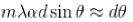(for small angles) where d is the width of the slit & in is an integer.
Since c = λv  one can relate the above equation to get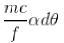Increasing frequency would decrease the angle thus, the fringes would get closer together. Increasing the frequency by a factor of 2 would decrease the separation by 2.

*Answer can only contain numeric values
QUESTION: 3

### A gas filled cell of length 0.5 cm is inserted in one arm of the michelson interferometer as shown in the figure below. The interferometer is illuminated by light of wavelength 500 nm. As the gas is evaluated from the cell, 40 fringes cross a point in the field of view. Find the refractive index of this gas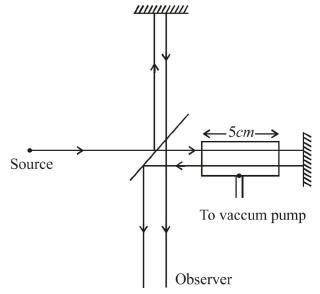Solution:

An effective path change of A produces a fringe shift. Thus, the interferometer formula is similar to the interference formula at normal incidence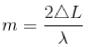Thus,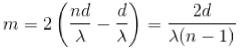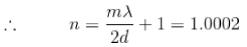*Answer can only contain numeric values
QUESTION: 4

Blue light of wavelength 480 nm is most strongly reflected from a thin film of oil on a glass slide when viewed near normal incidence. Assuming that the index of refraction of the oil is 1.2 & that of glass is 1.6, what is the minimum thickness of the oil film in nm (other than zero)?

Solution:

Assuming the boundaries are vacuum-oil, oil-glass, then there will be a phase shift at the vacuum oil boundary since the refractive index of vacuum nvac < noil  & another phase shift at the oil glass boundary because noil nglass That tells us that constructive interference happens for integer wavelength mλ.
Light crosses a distance d in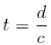but since its speed is lower in oil,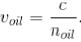Then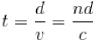∴ The condition for constructive interference becomes 2 noild = mλ
The next minimum thickness other than zero is when m = -1
So,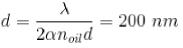*Answer can only contain numeric values
QUESTION: 5

In a double slit interference experiment d is the distance between the centres of the slits and w is the width of each slit. Find the minimum integer ratio d/ω for which an interference maximum of incident plane waves on a distant screen will be missing.

Solution:

We need a minimum of the single slit diffraction to coincide with a maximum of the double slit interference pattern (which result in a missing interference maximum)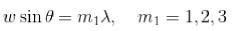[angulars location of minima of single slit diffraction]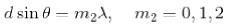[Angular locations of the maxima of double slit interference]
Ratio of 2 equations gives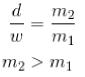So, minimum integer ratio is 2/1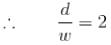*Answer can only contain numeric values
QUESTION: 6

It is necessary to coat a glass lens with a non reflecting layer if the wavelength of the light in the coating is λ, the best choice is a layer of material having an index of  refraction between those of glass & air and a thickness of what fraction of  λ ?

Solution:

In order for the thin film layer to be non reflecting, it must cancel the reflected wavelength as in a destructive interference the change in wavelength as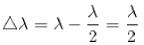Since, the wave changes phase by π at the interface below air and the coating, and again at the second interface below coating and glass
(Assume nair < ncoating < nglass). Destructive interference is thus given by a half integer wavelength change mλ/2 where the smallest change is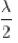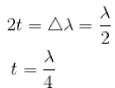*Answer can only contain numeric values
QUESTION: 7

Consider two horizontal glass plates with a thin film of air between them. Find the minimum value of the thickness of the film in nm of air for which the film as seen by the reflected light will appear bright if it is illuminated normally from above by blue light of wavelength 485 nm?

Solution:

For a thin film of thickness t, one can easily find the condition for interference phenomenon. Since the light has to travel approximately 2t to get back to the original incidence interface, one has 2t = mλ. However, since the light changes phase at the interface between air & glass, the condition for constructive interference becomes,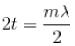Minimum thickness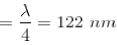*Answer can only contain numeric values
QUESTION: 8

Two coherent monochromatic light beams of intensities l and 4l are superposed. What is the minimum possible intensities in the resulting beam?

Solution:

In case of interference,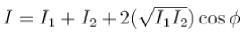So / will be maximum when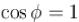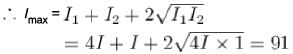And / will be minimum when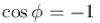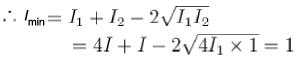*Answer can only contain numeric values
QUESTION: 9

The screen of a pinhole camera is at a distance D from the pinhole. Which has a diameter d the light has an effective wavelength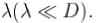For what value of d in terms of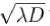will the image be sharpest

Solution:

The key equation involved is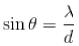For small angles,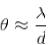This blur is due to both the size of the hole and the diffraction, the arc length of the diffraction is approximately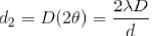where the factor of 2 comes from the fact that the arc length angle is symmetrical about the diffraction axis, thus twice the diffraction angle.
Define Blur equation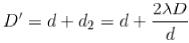taking the first derivative, w.r.t.
to d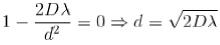The ratio of a intensities of light proceeding from the slits,